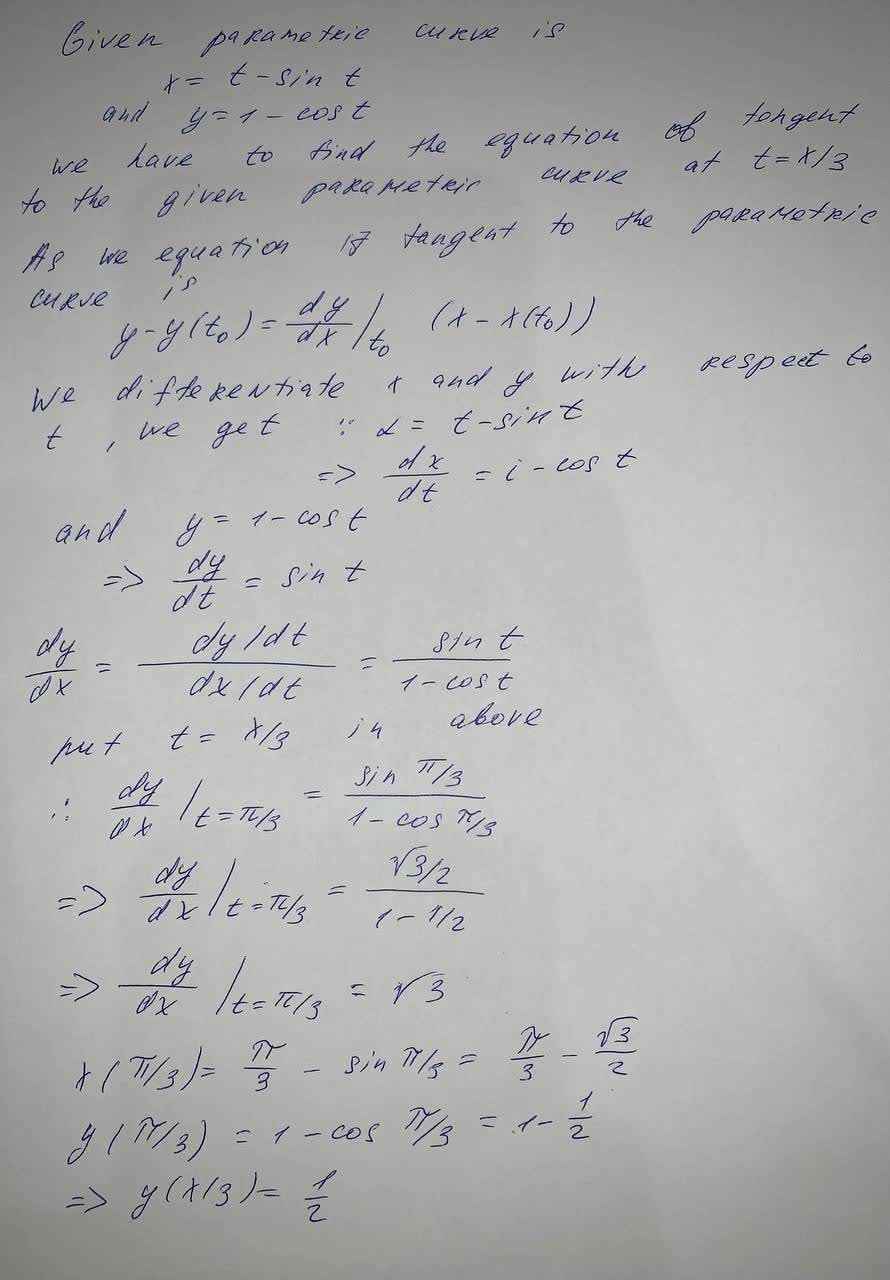In polar coordinates r^{2} = -6r\sin \theta equation in cartesian coordinates Find the equation and plot it.Haven 2021-09-05 Answered
In polar coordinates $$\displaystyle{r}^{{{2}}}=-{6}{r}{\sin{\theta}}$$ equation in cartesian coordinates
Find the equation and plot it.

• Questions are typically answered in as fast as 30 minutes

Solve your problem for the price of one coffee

• Math expert for every subject
• Pay only if we can solve itStrycharzT

To find the equation